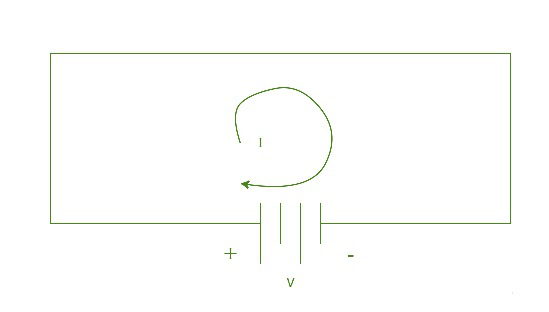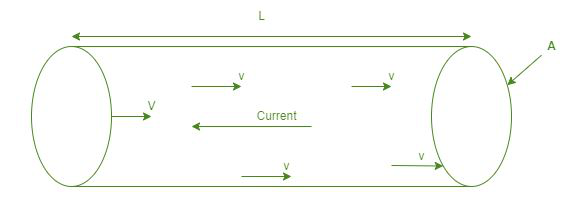Open in App
Not now

# Relation between Mobility and Electric Current

• Last Updated : 02 Sep, 2022

Mobility is correctly defined as the value of the drift velocity per unit of electric field strength. Hence, the quicker the particle moves at a specific electric field strength, the vast the mobility is. The mobility of any particular type of particle in a stated solid may differ with temperature. It is indicated by ‘μ’. It is expressed in m2/Vs.

### Electric current

An electric current is a course of charged particles, like electrons or ions, operating through an electrical conductor. It is measured as the net rate of flow of electric charge through a plane or into a control capacity. The symbol for electric current is ‘I’. It is expressed in ampere or ampere.From Ohm’s law

I = V/R

Where,

• I is the current flowing in the coil in amperes,
• V is the voltage in the coil in volts, and
• R is the resistance in ohms.### Relation between mobility and electric current

As we all know, the average velocity obtained by the charged particles in a conductor because of an electric field is called the Drift velocity. When a voltage is applied across the conductor, loose electrons acquire velocity in the opposite way of the electric field. Hence, there will be a small drift velocity. The equation to calculate drift velocity is,

Vd = I/neA

Where,

• I = current
• e = electron’s charge
• A = area
• n = free density of electron

The equation of mobility is given as,

μe = Vd/E

Where,

• μ = mobility
• E = electric field.

Derivation

From Newton’s second law,

F = ma

⇒ a = F/me*

Where

F = Force exerted by the electric field, a = acceleration between collisions, me* = effective mass of an electron.

We know the force on an electron is -eE

Therefore, a = -eE/m

And drift velocity Vd = aTc

= -eTcE/m

Here, Tc = mean free velocity.

We want to know how the drift velocity changes with the electric field, so we combine the loose terms together to get

Vd = -μeE

Where

μe = eTc/me*

For holes, Vd = μhE

Where μh = eTc/mh*

Note: Electron mobility and hole mobility both are positive. To account for the minus charge, a minus sign is added for electron drift velocity.

1. In an electrolyte, the charge carriers are both positive and negative ions.
2. In an ionized gas, the charge carriers are electrons and positive ions.

Key points

1. The mobility of a charge carrier is the mean velocity through which the carrier passes towards the positive tip of the conductor beneath the related potential difference.
2. The mobility of electrons, even as a charge carrier, is higher than in holes. Mobility is also defined as the capability to progress freely.
3. The electron in a conductor moves with a Fermi velocity, followed by zero average velocity. If we apply voltage, which will add to this net velocity, drift is formed.

### Sample Questions

Question 1: A current I flows through a uniform wire of diameter d when the electron drift velocity is V. the same current will flow through a wire of diameter d/4 made of the same material if the draft velocity of the electrons is:

Solution:

We know that,

Vd = I/neA

= I/[ne(πr2)]

From the given data

Vd= 1/neπ(4r)2

= (1/16)(1/neπr2)

Vd = Vd/16.

Question 2: Two wires, each having r as radius, with different materials, are connected together end to end. The densities of charge carriers in the two wires are in the ratio of 3:2, then the ratio of the drift velocity of electrons in the two wires is?

We know that the drift velocity is inversely proportional to the density of charge carriers.

Hence, the ratio of drift velocity is 2:3.

Question 3: A Cu wire has a cross-sectional area of 8 × 10-7 m2. The density of Cu is 8.5 × 1028 m-3. Calculate the mean drift velocity of the electrons through the wire when the current is 2A?

Solution:

Given,

I = 2A

n = 8.5 × 1028 m-3

A = 8 × 10-7 m2

Charge of electron e=1.6×10-19 Coulombs

We know that

Vd = I/neA

Vd  = 2/(8.5 × 1028 × 8 × 10-7 × 1.6 × 10-19)

Vd = 1.83 × 10-4 m/s.

Question 4: A charged particle having a drift velocity of 10*10-4 m/s in the electric field of 5 × 10-10 v/m, find the mobility?

Solution:

Given, Vd = 10 × 10-4 m/s

E = 5 × 10-10 v/m

Therefore, Mobility μ = |Vd|/E

μ = 10 × 10-4/5 × 10-10

μ = 2 × 106 m2/vs.

Question 5: A conducting wire has a radius of 15 mm, resistivity ρ = 1 × 10-8 ohm/m, and a current of 10 A is flowing. The drift velocity of a free electron is 1 × 10-3 m/s. Find the mobility of free electrons?

Solution:

We know that,

μ = Vd/E ⇒ Vd = μE

⇒ Vd = μ(V/l)

= μ.IR/l= μ.I.ρ.l/Al

⇒ Vd = μ.I.ρ/A

⇒ μ = Vd.A/Iρ

= (1 × 10-3 × π × 15 × 15)/(10 × 1 × 10-8)

Therefore, μ = 7.07 × 106 m2v/s.

Question 6: Cu contains 9 × 1012 free electrons/m3. A Cu wire of cross-sectional area 8 × 10-5 m2 carries a current of 2 A. Find the drift speed of the electron?

Solution:

From,

V = I/neA

V = 2/(9 × 1012 × 1.6 × 10-19 × 8 × 10-5)

⇒ V = 17.36 × 109 m/s.

Question 7: The drift velocity is 1 × 10-4 m/s when the current in a coil is 2 A. Find the drift velocity when the current becomes 4 A?

Solution:

Given, the initial current I = 2A

The initial drift velocity Vd = 1 × 10-4 m/s

Increased current I’ = 4 A

Therefore, increased drift velocity Vd‘ = ?

We know that,

I ∝ Vd

⇒ I’∝Vd

⇒ Vd‘/Vd = I’/I

⇒ Vd‘ = I’Vd/I

Hence, Vd‘ = 2 × 10-4 m/s.

My Personal Notes arrow_drop_up